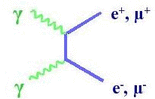H1Zeus - HERA Combined Results# MULTI-LEPTONS

Multi-lepton production at high transverse momentum has a very low cross section in the SM, dominated by γγ interactions. An anomalous production could sign the decay of new particles like exotic Higgs bosons.

### H1 + ZEUS combined results:

Multi-Leptons with High Transverse Momentum at HERA JHEP 0910:013 (2009)

(1) Mass distribution of the pair of the 2 highest PT leptons for the 5 topological channelsMass distribution of the pair of the 2 highest PT leptons for the 5 topological channels

(2) Distribution of the total transverse momentum carried by the leptons for the e+p collisions, the e-p collisions and the full data sampleDistribution of the total transverse momentum carried by the leptons for the e+p collisions, the e-p collisions and the full data sample

(3) Production cross section in the γγ domain as function of the lepton transverse momentum and the lepton pair massProduction cross section in the γγ domain as function of the lepton transverse momentum and the lepton pair mass